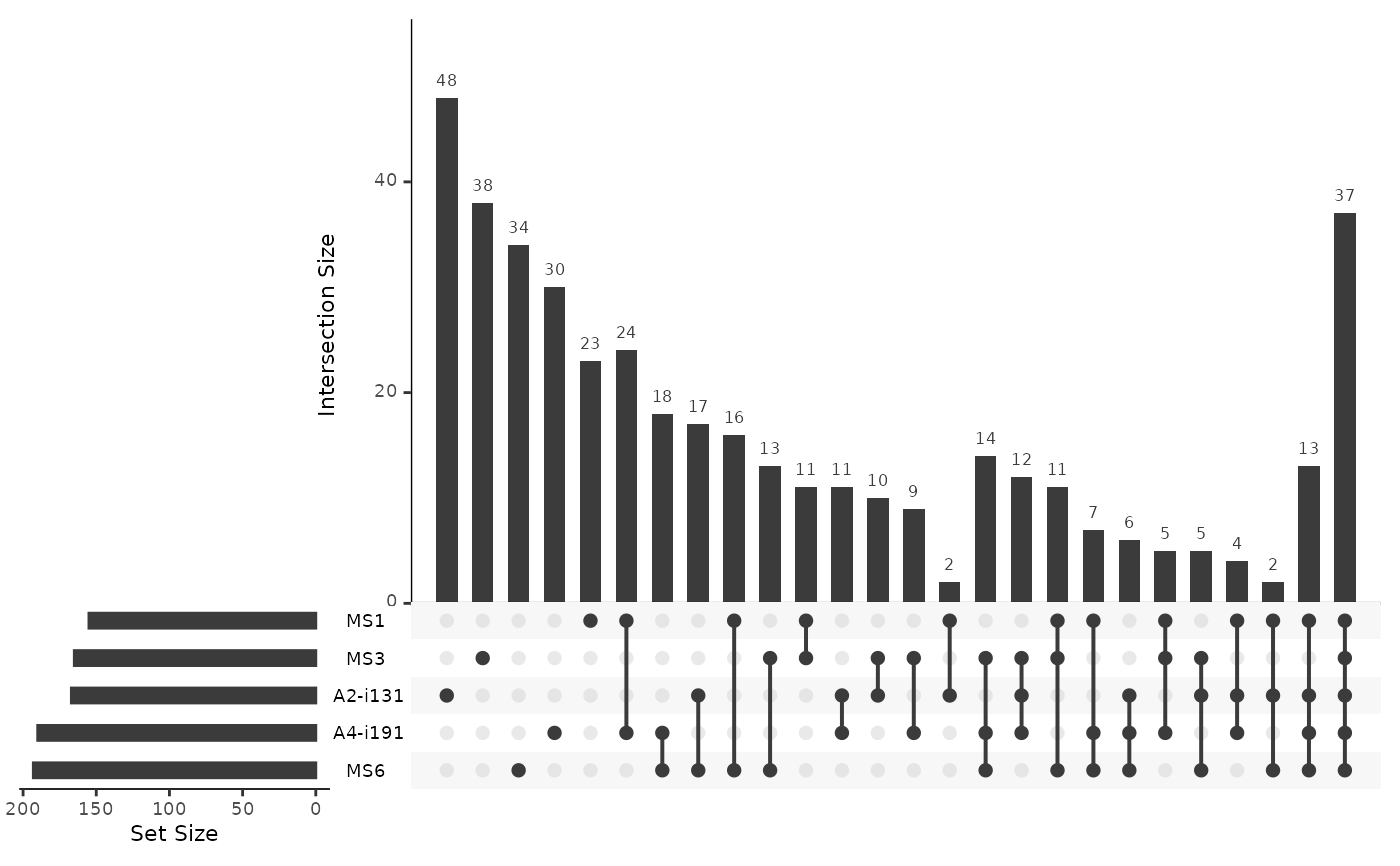Statistics of number of public clonotypes for each possible combinations of repertoires

pubRepStatistics(.data, .by = NA, .meta = NA)

## Arguments

.data

Public repertoire, an output from the pubRep function.

.by

Work in Progress.

.meta

Work in Progress.

## Value

Data frame with incidence statistics per sample.

## Examples

data(immdata)
immdata$data <- lapply(immdata$data, head, 2000)
pr <- pubRep(immdata\$data, .verbose = FALSE)
pubRepStatistics(pr) %>% vis()